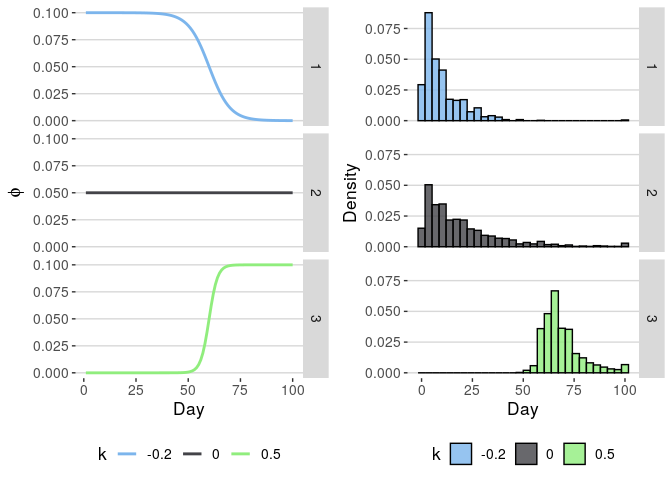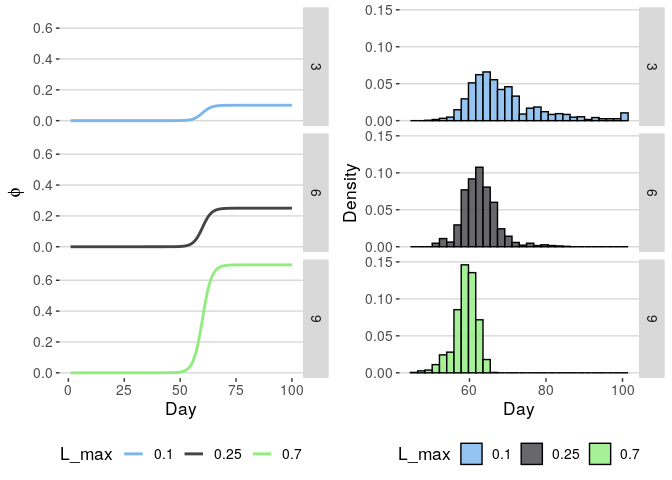# Introduction to the time-varying geometric distribution

#### Dec 04 , 2019

The time-varying geometric distribution has parameter $$\boldsymbol{\mathbf{\phi}}$$ and support from 1 to n + 1, where n =. If $$x\sim tvgeom(prob)$$, $$x = n + 1$$ when the event does not occur in the first $$n$$ trials. It has probability mass function

$f(x =1 \mid \boldsymbol{\mathbf{\phi}}) = \phi_x$ $f(x = i \mid \boldsymbol{\mathbf{\phi}}, 1<i\leq n ) = \phi_i\prod_{j = 1}^{i-1}(1 - \phi_j)$ $f(x = n + 1 \mid \boldsymbol{\mathbf{\phi}}) = \prod_{j = 1}^{n}(1 - \phi_j)$

## Description of the tvgeom distribution

The time-varying geometric distribution is derived from the geometric distribution, a discrete probability distribution used in econometrics, ecology, etc. Whereas the geometric distribution has a constant probability of success over time and has no upper bound of support, the time-varying geometric distribution has a probability of success that changes over time. Additionally, to accommodate situations in which the event can only occur in $$n$$ days, after which success can not occur, the time-varying geometric distribution is right-truncated (i.e. it has a maximum possible value determined by the length of $$\boldsymbol{\mathbf{\phi}}$$ above.

### In-depth example

First let’s load the packages we need.

library(tvgeom)
library(dplyr)
library(tidyr)
library(purrr)
library(magrittr)
library(ggplot2)
library(ggthemes)
library(gridExtra)

Next, let’s define a few functions, some wrappers, and the set of scenarios over which we hope to iterate in order to develop some sort of intuition.

# A logistic curve, which we can use to create a monotonically increasing or
# decreasing probability of success.
logistic <- function(n, x0, L_min, L_max, k, ...) {
(L_max - L_min) / (1 + exp(-k * (seq_len(n) - x0))) + L_min
}

# Wrappers.
get_phi <- function(data) {
data %>% pull(p_success) %>% c(1)
}
draw_from_tvgeom <- function(data, n_samples = 1000) {
rtvgeom(n_samples, get_phi(data))
}

# The total number of trials.
n_days <- 100

# Create an array of intuition-building scenarios. The time-varying probability
# of success (based upon which we will draw our samples) will depend entirely on
# the shape-controlling parameters of the curve for each scenario.
scenarios <- crossing(n = n_days,
x0 = 60,
L_min = 0,
L_max = c(.1, .25, .7),
k = c(-.2, 0, .5)
) %>%
mutate(scenario = as.character(1:n()))
Scenarios…
n x0 L_min L_max k scenario
100 60 0 0.10 -0.2 1
100 60 0 0.10 0.0 2
100 60 0 0.10 0.5 3
100 60 0 0.25 -0.2 4
100 60 0 0.25 0.0 5
100 60 0 0.25 0.5 6
100 60 0 0.70 -0.2 7
100 60 0 0.70 0.0 8
100 60 0 0.70 0.5 9

Next, let’s use some dplyr/purrr magic to develop data we can plot to show the effects of the various scenarios above.

# Calculate the probability of success for each scenario.
d_phi <- scenarios %>%
split(.$scenario) %>% map(~ do.call(logistic, .)) %>% bind_cols %>% mutate(day = 1:n()) %>% gather(scenario, p_success, -day) %>% left_join(scenarios) # On the basis of d_phi, make draws for new y's using rtvgeom. d_y <- d_phi %>% select(scenario, p_success) %>% split(.$scenario) %>%
map(~ draw_from_tvgeom(.)) %>%
bind_cols %>%
gather(scenario, y) %>%
left_join(scenarios)

# Plotting.
plot_param <- function(d_phi, d_y, parameter, subset = NULL) {

d1 <- d_phi %>%
mutate(focal_param = factor(get(parameter))) %>%
{if(!is.null(subset), filter_(., subset), .)}

p1 <- ggplot(d1) +
facet_grid(scenario ~ .) +
geom_line(aes_string(x = 'day', y = 'p_success', color = 'focal_param'),
size = 1.01) +
theme_hc(base_size = 13) +
scale_color_hc(name = parameter) +
labs(x = 'Day', y = expression(phi))

d2 <- d_y %>%
mutate(focal_param = factor(get(parameter))) %>%
{if(!is.null(subset), filter_(., subset), .)}
p2 <- ggplot(d2) +
facet_grid(scenario ~ .) +
geom_histogram(aes_string(x = 'y', y = '..density..', fill = 'focal_param'),
color = 'black', alpha = .8) +
theme_hc(base_size = 13) +
scale_fill_hc(name = parameter) +
labs(x = 'Day', y = 'Density')

grid.arrange(p1, p2, ncol = 2)

}

Plots of the resultsMax probability of success.